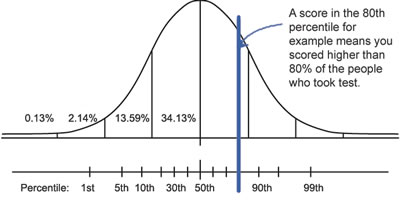# Difference between Percentile and Percentage

##### Key Difference: The two terms Percentile and Percentage are actually interrelated. A percentage is a calculation that depicts a ratio of something out of a 100. A percentile is a measure that indicates the value below which a given percentage of observations in a group of observations fall.Not only do Percentile and Percentage sound similar, but they even have similar meanings Both have something to do with mathematics and statistics, both fields that are challenging in itself to begin with.

The two terms Percentile and Percentage are actually interrelated. In order to understand percentile, one must first look at percentage. A percentage is a calculation that depicts a ratio of something on the scale of a 100. The term percentage literally means per cento, which is Latin for per hundred, i.e. out of a hundred. Basically, any number out of another number can be converted to out of a hundred and depicted as a percentage.

For example: in a class of 100, 45 students are male. Hence, 45% of the class is male. Here, 45% is equal to  45100, 45:100, or 0.45. Similarly, if in a class of 60, 36 students are male, then 3660 would come out to 0.6 or 60%. Basically, it means that if there are 60 students and 36 out of them are male, then if the class consisted of 100 students, then 60 of them would be male.

Now a percentile is something slightly different. While percentage is commonly used in nearly every field, percentile is used primarily in statistics. A percentile is a measure that indicates the value below which a given percentage of observations in a group of observations fall. Basically, it is sort of a percentage for a percentage.

For example: the 20th percentile is the value below which 20% of the observations may be found. The other 80% of the observations fall out of this category.It can also be said that percentile acts as a comparison for things. Let’s consider another example: a student falls in the 90th percentile. Hence, it can be said that out of 100, he did better than 90% of the other students, and that his scores are comparable to 10% of the class. This basically means that he is a really good student as he did better than most of the class.

It can also be said that percentage is absolute, regardless of what everyone else scored, the student’s score will be the same. For example: John scores 86 out of a 100, then his score is 86%. However, if there are 100 students in the class, and 70 of them scored less than 50%, then it can be said that John is in the 70% percentile as he did better than 70% of the class.

Comparison between Percentile and Percentage:

 Percentile Percentage Field Statistics Mathematics, as well as in nearly every other field. Description A percentile is a measure that indicates the value below which a given percentage of observations in a group of observations fall. A percentage is a calculation that depicts a ratio of something on the scale of a 100. Purpose Ranking as compared to others Out of a 100 Relativity Relative to other outside factors Absolute will be fixed regardless Formula cℓ +0.5 ƒi x 100%      N   Where cℓ is the count of all scores less than the score of interest, ƒi is the frequency of the score of interest, and N is the number of examinees in the sample. X x 100 Y   Where X is the number out of Y, which is the whole.
```Reference: Wikipedia (Percentage, Percentile, and Percentile Rank),
MathisFun, NWEA, BBC, Numerical Reasoning
Image Courtesy: bbc.co.uk, numericalreasoning.co.uk```